# JP2010033352A - Lane change alarm and program - Google Patents

## Info

Publication number
JP2010033352A
JP2010033352A JP2008195156A JP2008195156A JP2010033352A JP 2010033352 A JP2010033352 A JP 2010033352A JP 2008195156 A JP2008195156 A JP 2008195156A JP 2008195156 A JP2008195156 A JP 2008195156A JP 2010033352 A JP2010033352 A JP 2010033352A
Authority
JP
Japan
Prior art keywords
vehicle
lane change
evaluation value
plurality
means
Prior art date
Legal status (The legal status is an assumption and is not a legal conclusion. Google has not performed a legal analysis and makes no representation as to the accuracy of the status listed.)
Pending
Application number
JP2008195156A
Other languages
Japanese (ja)
Inventor
Chiyomi Miyajima
Yoshihiro Nishiwaki
Kazuya Takeda
Ryuta Terashima
Toshihiro Wakita

Original Assignee
Nagoya Univ
Toyota Central R&D Labs Inc

Priority date (The priority date is an assumption and is not a legal conclusion. Google has not performed a legal analysis and makes no representation as to the accuracy of the date listed.)
Filing date
Publication date
Application filed by Nagoya Univ, Toyota Central R&D Labs Inc, 国立大学法人名古屋大学, 株式会社豊田中央研究所 filed Critical Nagoya Univ
Priority to JP2008195156A priority Critical patent/JP2010033352A/en
Publication of JP2010033352A publication Critical patent/JP2010033352A/en
Pending legal-status Critical Current

• 238000004364 calculation methods Methods 0.000 claims abstract description 37
• 238000000034 methods Methods 0.000 description 15

## Images

•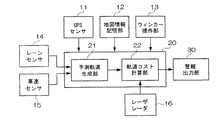•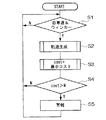•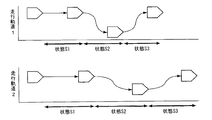•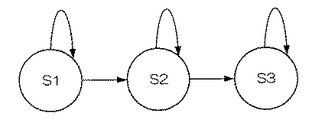••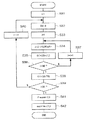••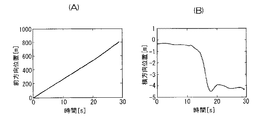## Abstract

<P>PROBLEM TO BE SOLVED: To prevent an accident when changing a lane by estimating the motion of not only the other vehicle but also one's own vehicle. <P>SOLUTION: A lane change alarm includes: an estimated trajectory generation part 21 for storing hidden Markov model parameters for dividing the behavior of the vehicle into a plurality of statuses and representing the statuses, and generating a plurality of estimated trajectories of the vehicle so as to maximize a simultaneous occurrence probability by use of the hidden Markov model parameters; a laser radar 16 for detecting the position of the vehicle relative to the other vehicle; a trajectory cost calculation part 22 for calculating the security evaluation value in a plurality of predetermined position relations with the other vehicle for each of the plurality of estimated trajectories on the basis of the detected position relative to the other vehicle, and calculating the safest evaluation value; and an alarm output part 30 for outputting an alarm when the calculated evaluation value does not satisfy predetermined security. <P>COPYRIGHT: (C)2010,JPO&INPIT

## Description

The present invention relates to a lane change warning device and a program.

Conventionally, a moving object collision prevention device for imaging the rear from a camera mounted on the own vehicle, detecting other vehicles from the image, and avoiding troubles related to collision and abnormal approach of both vehicles based on the detection result It is disclosed (see Patent Document 1).

The technology of Patent Document 1 observes the situation around a running vehicle using a TV camera, detects surrounding vehicles that may collide, and warns the driver of the vehicle based on the result. appear. Here, the above technique calculates the amount of movement of the feature points with respect to the interval between frames, detects the overtaking vehicle from an area having the same amount of movement, predicts the time for the overtaking vehicle to catch up with the own vehicle, An alarm is generated when is below the threshold.
JP 2000-285245 A

However, the technique of Patent Document 1 predicts only the movement of another vehicle based on the amount of movement of the feature point with respect to the interval between frames, and does not predict the movement of the host vehicle. For this reason, there is a problem that the possibility of collision between the host vehicle and another vehicle cannot be estimated correctly.

The present invention has been proposed to solve the above-described problems, and provides a lane change maintaining device and a program that can prevent an accident by predicting the movement of not only another vehicle but also the host vehicle. Objective.

The lane change alarm device according to the present invention stores a hidden Markov model parameter for expressing a behavior of a vehicle in a lane change with respect to at least one of a vehicle movement state, a vehicle operation amount, and a vehicle traveling environment in a plurality of states. And the vehicle movement state, the vehicle operation amount, the vehicle traveling environment, and the hidden Markov model parameters stored in the storage means, and the vehicle is configured to maximize the co-occurrence probability. A predicted trajectory generating means that generates a plurality of predicted trajectories, a relative position of the other vehicle detected by the other vehicle position detecting means, and a relative position of the other vehicle detected by the other vehicle position detecting means. Based on the specific position, safety evaluation values in a plurality of predetermined positional relationships with other vehicles are generated by the predicted trajectory generating means. An evaluation value calculation means for calculating the highest safety evaluation value and an alarm when the evaluation value calculated by the evaluation value calculation means does not satisfy a predetermined safety. Alarm output means for outputting.

The lane change alarm program according to the present invention is a program for causing a computer to function as each means of the lane change alarm device.

According to the above invention, a plurality of predicted trajectories of the vehicle are generated and generated so as to maximize the co-occurrence probability using the hidden Markov model parameters for representing the behavior of the vehicle in the lane change divided into a plurality of states. Further, safety evaluation values in a plurality of predetermined positional relationships with other vehicles are calculated for each of the plurality of predicted tracks, and the highest safety evaluation value is obtained. Then, an alarm is output when the evaluation value does not satisfy the predetermined safety.

Thereby, since the said invention predicts the track | orbit of a lane change and outputs a warning with respect to a driver when it is not a safe lane change, the accident at the time of a lane change can be prevented.

The lane change warning device and program according to the present invention can prevent an accident at the time of lane change by predicting not only other vehicles but also the movement of the host vehicle.

Hereinafter, preferred embodiments of the present invention will be described in detail with reference to the drawings.

FIG. 1 is a block diagram showing a configuration of a lane change warning device according to an embodiment of the present invention. The lane change warning device is mounted on a vehicle capable of traveling in the present embodiment, but may be mounted on, for example, a driving simulator.

The lane change warning device includes a GPS (Global Positioning System) sensor 11 that detects an absolute position (latitude and longitude) based on a signal from a positioning satellite, a map information storage unit 12 that stores map information, and a direction indicator lamp. And a blinker operation unit 13 for performing an operation of turning on the blinker.

Further, the lane change alarm device includes a lane sensor 14 that detects a lane in which the vehicle is traveling, a vehicle speed sensor 15 that detects a vehicle speed (vehicle speed), a laser radar 16 that detects a peripheral object by a reflected wave of laser irradiation, and a lane change. Are provided with an arithmetic processing unit 20 that performs arithmetic processing for and an alarm output unit 30 that outputs an alarm.

The arithmetic processing unit 20 includes a central processing unit (CPU) and the like, and functionally, a predicted trajectory generation unit 21 that generates predicted trajectories, and a trajectory cost that calculates the cost of each predicted trajectory. And a calculation unit 22.

The lane change warning device configured as described above performs the following processing in order to issue a warning for dangerous lane changes.

(Main processing routine)
FIG. 2 is a flowchart showing the main processing routine.

In step S1, the arithmetic processing unit 20 reads the absolute position of the host vehicle detected by the GPS sensor 11 and the map information of the surrounding area from the map information storage unit 12, and determines whether the host vehicle is traveling on an automobile road. To do. Furthermore, the arithmetic processing unit 20 determines whether or not a winker operation has been performed based on a signal from the winker operation unit 13. Then, the arithmetic processing unit 20 waits until it is determined that the vehicle has traveled on the car road and the winker operation has been performed, and the vehicle has traveled on the car road and the winker operation is performed. If it is determined that it has been, the process proceeds to step S2.

In step S2, the arithmetic processing unit 20 generates a plurality of predicted trajectories of the host vehicle based on the detection results of the lane sensor 14 and the vehicle speed sensor 15, and proceeds to step S3. Details of step S2 will be described later.

In step S3, the arithmetic processing unit 20 calculates the cost for each of the plurality of predicted trajectories generated in step S2, and substitutes the minimum cost, which is the minimum value of the calculated cost, into the variable cost (cost = Minimum cost), the process proceeds to step S4. Details of step S4 will be described later.

In step S4, the arithmetic processing unit 20 determines whether or not the variable cost exceeds the threshold value W. If it exceeds, the processing proceeds to step S5, and if not, returns to step S1.

In step S5, the arithmetic processing unit 20 controls the alarm output unit 30 to output an alarm, and returns to step S1 again. Thereby, the warning output unit 30 outputs a warning by sound or image.

(Step S2: Generation of predicted trajectory)
In step S2 described above, the predicted trajectory generation unit 21 of the arithmetic processing unit 20 generates a plurality of predicted trajectories using a previously stored hidden Markov model (HMM). In this embodiment, the predicted trajectory has three states (a state S1 in which the driver plans a lane change, a state S2 in which the driver is moving from the traveling lane to the overtaking lane, and a movement from the overtaking lane to the original traveling lane. State S3).

Here, the driver does not necessarily have the same behavior even if the situation is the same. However, this behavioral uncertainty can be considered as a stochastic process and modeled. In the present embodiment, a vehicle operation in an arbitrary environment, a vehicle state, and a change in the time direction thereof are regarded as a hidden Markov model, and the behavior of the vehicle in a specific scene is described.

FIG. 3 is a diagram showing a predicted trajectory (traveling trajectory) and states S1, S2, and S3. FIG. 4 is a state transition diagram of the three states S1 to S3 of the predicted trajectory. As shown in FIG. 3, all predicted trajectories are divided into state 1, state 2, and state 3.

FIG. 4 is a model of the behavior of a vehicle during a lane change by a three-state Left-to-Right type HMM. Here, the transition is made in the order of the states S1, S2, and S3, and there is no transition from the state S1 to S3 beyond S2. Further, from each state S, ot = [ct, Δct, ΔΔct] representing the vehicle motion state, the vehicle operation amount, and the vehicle traveling environment, respectively.
Is output as a label sequence. Where ct is a determinant,
ct = [y, x]
It is. Note that y is the distance in the left-right direction from the right or left lane of the vehicle to the reference position of the vehicle, and x is the vehicle speed of the host vehicle in the front-rear direction (straight direction). That is, from each state S, the lane position (the distance in the horizontal direction from the vehicle right or left lane to the vehicle reference position) y, the vehicle speed x in the front-rear direction, and the primary difference Δct and the secondary difference ΔΔct are obtained. Is output. Note that the label series is not limited to those described above, and it is sufficient that there is at least one of the parameters of the vehicle motion state, the vehicle operation amount, and the vehicle travel environment.

The HMM has a state transition probability, a symbol output probability, and an initial state probability as parameters. Here, since the state duration can take any time according to the state transition probability, various vehicle behaviors can be obtained from the HMM model, for example, as shown in FIG. That is, if the HMM is determined based on the behavior of an arbitrary driver, the behavior of the vehicle at the time of the lane change can be reproduced probabilistically.

Therefore, the predicted trajectory generation unit 21 probabilistically determines the time required for the vehicle to perform a lane change (hereinafter referred to as “lane change time”), and then calculates the state stop time in each state expressing the lane change time length. Each is calculated using the HMM, and the trajectory of each state is generated from the state stop time. Specifically, the next predicted trajectory generation routine is executed.

FIG. 5 is a flowchart showing a predicted trajectory generation routine.

In step S11, the predicted trajectory generation unit 21 sets 1 to the time index number i (i = 1), and proceeds to step S12. The time index number i is an index number given to each different lane change time, and is 1 to N1 in this embodiment.

In step S12, the predicted trajectory generation unit 21 generates a Gaussian random number based on the average time μT required for the lane change by the reference driver and the standard deviation σT of the time required for the lane change by the reference driver. Note that μT and σT are parameters obtained in advance by experiments or simulations. Then, the predicted trajectory generation unit 21 calculates the generated Gaussian random number as the lane change time T (i), and proceeds to step S13. The predicted trajectory generation unit 21 generates gauss random numbers in this way, thereby calculating various variations of lane change times.

In step S13, the predicted trajectory generation unit 21 sets 1 for the deformation index number j (j = 1), and proceeds to step S14. The modified index number j is an index number given to each different predicted trajectory at a certain lane change time T (i), and is 1 to N2 in this embodiment.

In step S14, the predicted trajectory generation unit 21 sets 1 to the state index number k (k = 1), and proceeds to step S15. Note that the state index number k is an index number indicating the numerical part of the states S1 to S3 shown in FIG. 4, and is 1 to N3 (= 3) in this embodiment.

In step S15, the predicted trajectory generation unit 21 calculates the state duration d (k) according to the following equation, and proceeds to step S16.

Note that μ qk is an average state retention time in the state Sk, and x qk is a random variable according to a uniform distribution (0, 1) in the state Sk.

In step S16, the predicted trajectory generation unit 21 determines whether or not the state index number is less than N3 (k <N3 = 3). If the determination is affirmative, the process proceeds to step S18. If the determination is negative, step S17 is performed. Proceed to

In step S17, the predicted trajectory generation unit 21 increments the state index number k (k ← k + 1), and returns to step S15. As described above, when Steps S15 to S17 are repeatedly executed, the respective state duration times d (k) of the states S1 to S3 in the lane change time T (i) are calculated.

In step S18, the predicted trajectory generation unit 21 uses the state durations d (k) of the states S1 to S3 at the lane change time T (i) to maximize the likelihood of the lane position and the forward vehicle speed train. Such a trajectory (maximum likelihood path) is generated, and this trajectory is substituted into the variable tr (i, j), and the process proceeds to step S19. Specifically, when the vector of the lane position and the forward vehicle speed train is represented by c, the vector including the primary and secondary differences is represented by o, and the state transition sequence and the lane change time obtained from the state duration are given, The likelihood under the parameter λ of the HMM learned in advance can be expressed as p (o | d (k), T (i), λ). The maximum likelihood path is obtained by calculating a series of c that maximizes the log likelihood, that is, the following expression.

In step S19, the predicted trajectory generation unit 21 determines whether or not the deformation index number j is less than N2 (j <N2). If the determination is affirmative, the process proceeds to step S21. If the determination is negative, the process proceeds to step S20. move on.

In step S20, the predicted trajectory generation unit 21 increments the deformation index number j (j ← j + 1), and returns to step S14. As described above, when Steps S14 to S20 are repeatedly executed, N2 predicted trajectories (maximum likelihood paths) in the lane change time T (i) are generated.

In step S21, the predicted trajectory generation unit 21 determines whether or not the time index number i is less than N1 (i <N1). If the determination is affirmative, this routine is terminated. If the determination is negative, step S22 is performed. Proceed to

In step S22, the predicted trajectory generation unit 21 increments the time index number i (i ← i + 1), and returns to step S12. When Steps S1 to S22 are repeatedly executed, N2 predicted trajectories (maximum likelihood paths) are generated for each of N1 lane change times T (i), and a total (N1 × N2) predicted A trajectory is generated and the routine ends.

As for algorithms related to HMM, Tokuda et al., “SPEECH PARAMETER GENERATION ALGORITHMS FOR HMM-BASED SPEECH SYNTHESIS”, Proc of IEEE International Conference on Acoustics, Speech, and Signal Processing (ICASSP), vol.3, pp.1315-1318, June 2000.

(Step S3: Cost calculation of predicted trajectory)
In step S3, the trajectory cost calculation unit 22 of the arithmetic processing unit 20 executes the following trajectory cost calculation routine in order to calculate the cost for evaluating each predicted trajectory generated in step S2.

FIG. 6 is a flowchart showing the orbit cost calculation routine.

In step S31, the trajectory cost calculation unit 22 sets the index number i to 1 (i = 1), and proceeds to step S32.

In step S32, the trajectory cost calculation unit 22 initializes the variable C (i), and proceeds to step S33. C (i) is a variable for storing a cost value for evaluating the appropriateness of the lane change time T (i) at the index number i. C (i) is a time average value of the traveling locus of N3 (= 3) variations that lane change at the lane change time T (i), as will be described in detail later.

In step S33, the trajectory cost calculation unit 22 sets the deformation index number j to 1 (j = 1), and proceeds to step S34.

In step S34, the trajectory cost calculation unit 22 calculates the cost of the predicted trajectory tr (i, j) based on the detection result of the laser radar 16 shown in FIG. In the present embodiment, the trajectory cost calculation unit 22 calculates a cost function for each of the following four unsafe cases.

FIG. 7 is a diagram showing four unsafe cases, where (A) is when the host vehicle is too close to the preceding vehicle (case 1), and (B) is when the host vehicle is too close to the adjacent front vehicle (case 2). , (C) shows a case where the vehicle is too close to the adjacent rear vehicle (Case 3), and (D) shows a case where the vehicle is running parallel to the adjacent front vehicle (Case 4). In the figure, V0 indicates the host vehicle, and at the time of lane change, four unsafe scenes (A) to (D) are assumed from the relative positional relationship with surrounding vehicles. Cases 1 to 3 are essential, but case 4 is optional.

Here, the detection result of the laser radar 16 used in the cost calculation is a distance F *, i, in the front-rear direction (traveling direction) to the preceding vehicle V1, the adjacent front vehicle V2, or the adjacent rear vehicle V3 with respect to the own vehicle V0 . j [m] is a distance R *, i, j [m] in the left-right direction (lateral direction) from the host vehicle V0 to the preceding vehicle V1 or the adjacent front vehicle V2. Note that p is a number indicating a vehicle for cost calculation. In case 1, p = 1, in cases 2 and 4, p = 2, and in case 3, p = 3.

Specifically, in the case 1, the trajectory cost calculation unit 22 performs the following comparison.

In the case 2, the track cost calculation unit 22 performs the following comparison.

In the case 3, the track cost calculation unit 22 performs the following comparison.

In the case 4, the track cost calculation unit 22 performs the following comparison.

When these comparison expressions hold, the distance between the host vehicle and the other vehicle is less than a predetermined value in each case, and the vehicle is approaching unsafely. In the cost calculation, it is assumed that the vehicle speeds of the surrounding vehicles are constant from the time when generation of the predicted trajectory is started. Then, the trajectory cost calculation unit 22 substitutes the number for which the above comparison expression is satisfied into the variable c (i, j) as the cost of tr (i, j), and proceeds to step S35.

In step S35, the trajectory cost calculation unit 22 adds c (i, j) to C (i) (C (i) = C (i) + c (i, j)), and proceeds to step S36.

In step S36, the trajectory cost calculation unit 22 determines whether the deformation index number j is less than N2 (j <N2). If the determination is affirmative, the process proceeds to step S38. If the determination is negative, the process proceeds to step S37.

In step S37, the trajectory cost calculation unit 22 increments the deformation index number j (j ← j + 1), and returns to step S34. As described above, when Steps S34 to S37 are repeatedly executed, N2 costs in the lane change time T (i) are added.

In step S38, the trajectory cost calculation unit 22 normalizes C (i) with the lane change time T (i) (C (i) / T (i)), and substitutes the obtained value into C (i). Then, the process proceeds to step S39. C (i) obtained here is the cost of the lane change time T (i).

In step S39, the trajectory cost calculation unit 22 determines whether i is less than N1. If the determination is affirmative, the process proceeds to step S41. If the determination is negative, the process proceeds to step S40.

In step S40, the trajectory cost calculation unit 22 increments i (i ← i + 1), and returns to step S32. Then, when Steps S32 to S40 are repeatedly executed, each cost in N1 lane change times T (i) is calculated.

In step S41, the trajectory cost calculation unit 22 sets the time index number i of the lane change time T (i) at which the lane change is performed at the lowest cost among the N1 lane change times T (i). The variable i * is stored (i * = argmin C (i)), and the process proceeds to step S42.

In step S42, the trajectory cost calculation unit 22 stores the cost for the predicted trajectory tr (i * , j) giving the minimum cost in the lane change time T (i * ) in the variable cost (cost = min c (i * , j)), this routine is terminated.

Then, the trajectory cost calculation unit 22 determines whether or not the variable cost exceeds the threshold value W (step S4 in FIG. 2), and if it exceeds, controls to output an alarm to the alarm output unit 30. (Step S5).

FIG. 8 is a diagram illustrating an example of predicted trajectories in (A) the front-rear direction and (B) the lateral direction. As shown in the figure, for example, a trajectory that moves at a constant speed in the front-rear direction and smoothly moves in the lateral direction is generated.

As described above, the lane change warning device predicts the movement of the own vehicle by generating a predicted trajectory at the time of a typical lane change of a normative driver in an arbitrary driving environment, and not only a passing vehicle but also a surrounding vehicle. By calculating the trajectory cost in consideration of the physical positional relationship, it is possible to precisely estimate the possibility of collision and issue a warning.

That is, the lane change warning device predicts the operation at the time of a lane change in advance, so that when the driver tries to make an unsafe lane change, the driver is informed of the danger, so an accident can be prevented.

It should be noted that the present invention is not limited to the above-described embodiment, and it is needless to say that the present invention can also be applied to a design modified within the scope described in the claims.

It is a block diagram which shows the structure of the lane change warning device which concerns on embodiment of this invention. It is a flowchart which shows a main process routine. It is a figure which shows a prediction track | orbit (running track) and states S1, S2, and S3. It is a state transition diagram of three states S1-S3 of a prediction orbit. It is a flowchart which shows a prediction trajectory generation routine. It is a flowchart which shows a track cost calculation routine. It is a figure which shows four unsafe cases. It is a figure which shows an example of the prediction track | orbit of (A) front-back direction and (B) horizontal direction.

Explanation of symbols

DESCRIPTION OF SYMBOLS 11 GPS sensor 12 Map information memory | storage part 13 Winker operation part 14 Lane sensor 15 Vehicle speed sensor 16 Laser radar 20 Arithmetic processing unit 21 Prediction track generation part 22 Track cost calculation part 30 Alarm output part

## Claims (5)

1. Storage means for storing hidden Markov model parameters for dividing the behavior of the vehicle in a lane change with respect to at least one of a vehicle motion state, a vehicle operation amount, and a vehicle traveling environment into a plurality of states;
Based on at least one of the vehicle motion state, the vehicle operation amount, the vehicle travel environment, and a hidden Markov model parameter stored in the storage means, a plurality of the vehicle A predicted trajectory generating means for generating a predicted trajectory;
Other vehicle position detection means for detecting a relative position of the vehicle with respect to the other vehicle;
Based on the relative position with respect to the other vehicle detected by the other vehicle position detection means, safety evaluation values in a plurality of predetermined positional relationships with the other vehicle are generated by the predicted trajectory generation means. An evaluation value calculation means for calculating the highest safety evaluation value for each of a plurality of predicted trajectories,
An alarm output means for outputting an alarm when the evaluation value calculated by the evaluation value calculation means does not satisfy a predetermined safety;
Lane change alarm device with
2. The predicted trajectory generating means generates a lane change time based on the lane change average time and the standard deviation of the reference driver, and based on the HMM model parameters stored in the storage means for the generated lane change time. The lane change warning device according to claim 1, wherein the duration of each state is calculated, and a plurality of predicted trajectories of the vehicle are generated so as to maximize the co-occurrence probability using the duration of each state.
3. The evaluation value calculation means calculates, as the evaluation value, the number of front and rear distances with other vehicles in the front-rear direction and the number of straight distances with other vehicles that are less than a predetermined threshold value, respectively. Item 3. Lane change alarm device according to item 2.
4. Computer
Storage means for storing hidden Markov model parameters for dividing the behavior of the vehicle in a lane change with respect to at least one of a vehicle motion state, a vehicle operation amount, and a vehicle traveling environment into a plurality of states;
Based on at least one of the vehicle motion state, the vehicle operation amount, the vehicle travel environment, and a hidden Markov model parameter stored in the storage means, a plurality of the vehicle A predicted trajectory generating means for generating a predicted trajectory;
Other vehicle position detection means for detecting a relative position of the vehicle with respect to the other vehicle;
Based on the relative position with respect to the other vehicle detected by the other vehicle position detection means, safety evaluation values in a plurality of predetermined positional relationships with the other vehicle are generated by the predicted trajectory generation means. An evaluation value calculation means for calculating the highest safety evaluation value for each of a plurality of predicted trajectories,
An alarm output means for outputting an alarm when the evaluation value calculated by the evaluation value calculation means does not satisfy a predetermined safety;
Lane change warning program to make it function.
5. Computer
The lane change warning program for functioning as each means of the lane change warning device of any one of Claims 1-3.
JP2008195156A 2008-07-29 2008-07-29 Lane change alarm and program Pending JP2010033352A (en)

## Priority Applications (1)

Application Number Priority Date Filing Date Title
JP2008195156A JP2010033352A (en) 2008-07-29 2008-07-29 Lane change alarm and program

## Applications Claiming Priority (1)

Application Number Priority Date Filing Date Title
JP2008195156A JP2010033352A (en) 2008-07-29 2008-07-29 Lane change alarm and program

## Publications (1)

Publication Number Publication Date
JP2010033352A true JP2010033352A (en) 2010-02-12

# Family

## Family Applications (1)

Application Number Title Priority Date Filing Date
JP2008195156A Pending JP2010033352A (en) 2008-07-29 2008-07-29 Lane change alarm and program

## Country Status (1)

JP (1) JP2010033352A (en)

## Cited By (6)

* Cited by examiner, † Cited by third party
Publication number Priority date Publication date Assignee Title
CN102568200A (en) * 2011-12-21 2012-07-11 辽宁师范大学 Method for judging vehicle driving states in real time
CN102819259A (en) * 2011-06-09 2012-12-12 清华大学 Functional security verification method for safety instrument based on Markov process
KR101480652B1 (en) 2013-12-11 2015-01-09 현대자동차주식회사 Lane change control apparatus and control method of the same
CN106205213A (en) * 2014-12-30 2016-12-07 江苏理工学院 A kind of boats and ships trajectory predictions method
WO2017002258A1 (en) * 2015-07-02 2017-01-05 三菱電機株式会社 Route prediction device
KR101795250B1 (en) * 2016-05-03 2017-11-07 현대자동차주식회사 Path planning apparatus and method for autonomous vehicle

## Cited By (10)

* Cited by examiner, † Cited by third party
Publication number Priority date Publication date Assignee Title
CN102819259A (en) * 2011-06-09 2012-12-12 清华大学 Functional security verification method for safety instrument based on Markov process
CN102568200A (en) * 2011-12-21 2012-07-11 辽宁师范大学 Method for judging vehicle driving states in real time
CN102568200B (en) * 2011-12-21 2015-04-22 辽宁师范大学 Method for judging vehicle driving states in real time
KR101480652B1 (en) 2013-12-11 2015-01-09 현대자동차주식회사 Lane change control apparatus and control method of the same
CN106205213A (en) * 2014-12-30 2016-12-07 江苏理工学院 A kind of boats and ships trajectory predictions method
WO2017002258A1 (en) * 2015-07-02 2017-01-05 三菱電機株式会社 Route prediction device
WO2017002441A1 (en) * 2015-07-02 2017-01-05 三菱電機株式会社 Route prediction device
JPWO2017002441A1 (en) * 2015-07-02 2017-09-14 三菱電機株式会社 Route prediction device
KR101795250B1 (en) * 2016-05-03 2017-11-07 현대자동차주식회사 Path planning apparatus and method for autonomous vehicle
US10259458B2 (en) 2016-05-03 2019-04-16 Hyundai Motor Company Path planning apparatus and method for autonomous vehicle

## Similar Documents

Publication Publication Date Title
US10268909B2 (en) Systems and methods for near-crash determination
JP6232004B2 (en) Method for performing risk-based traffic scene analysis by advanced driver assistance system, and vehicle equipped with the system for performing the analysis
US9308919B2 (en) Composite confidence estimation for predictive driver assistant systems
Tawari et al. Looking-in and looking-out vision for urban intelligent assistance: Estimation of driver attentive state and dynamic surround for safe merging and braking
US10239523B2 (en) Route evaluation device
DE102016212751A1 (en) abbiegevorhersagen
JP6650214B2 (en) Method and system for post-collision maneuver planning, and vehicle equipped with the system
JP6375221B2 (en) Method and system for predicting the movement behavior of a target traffic object
JP6684714B2 (en) Method and system for vehicle driver assistance
CN106952471B (en) Prediction of driver intent at an intersection
US9129519B2 (en) System and method for providing driver behavior classification at intersections and validation on large naturalistic data sets
US8788134B1 (en) Autonomous driving merge management system
DE102013113572B4 (en) A method of identifying potential hazards in the environment of a vehicle
US9400897B2 (en) Method for classifying parking scenarios for a system for parking a motor vehicle
US9424468B2 (en) Moving object prediction device, hypothetical movable object prediction device, program, moving object prediction method and hypothetical movable object prediction method
Schubert et al. Situation assessment for automatic lane-change maneuvers
US10332403B2 (en) System and method for vehicle congestion estimation
Kumar et al. Learning-based approach for online lane change intention prediction
US8903588B2 (en) Method and system for predicting movement behavior of a target traffic object
US9889858B2 (en) Confidence estimation for predictive driver assistance systems based on plausibility rules
US8112225B2 (en) Method and system for collision avoidance
Aoude et al. Driver behavior classification at intersections and validation on large naturalistic data set
Angkititrakul et al. On the use of stochastic driver behavior model in lane departure warning
Berndt et al. Continuous driver intention recognition with hidden markov models
US9224299B2 (en) Cruise control plan evaluation device and method